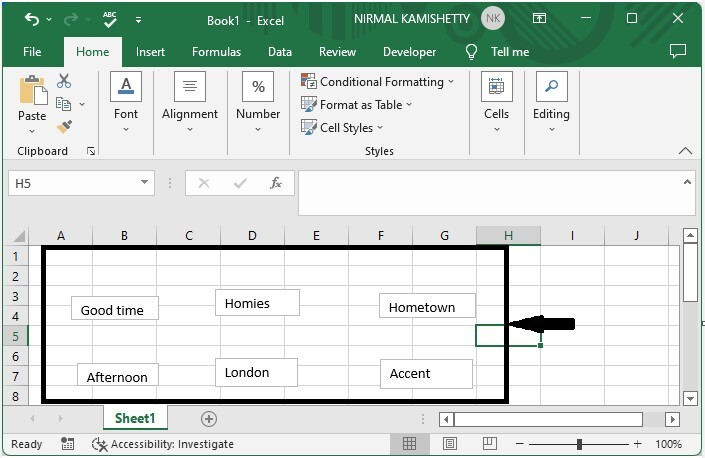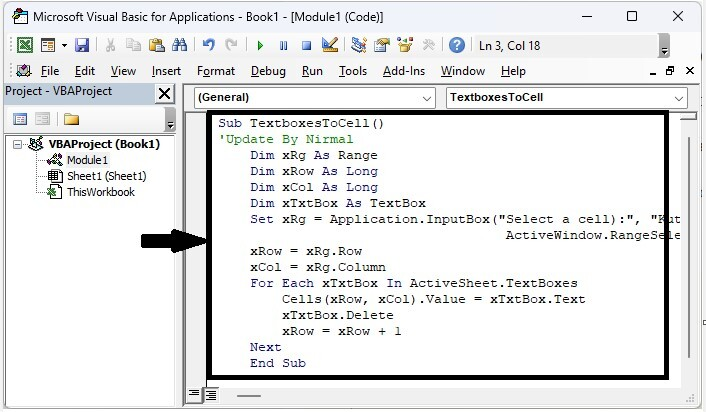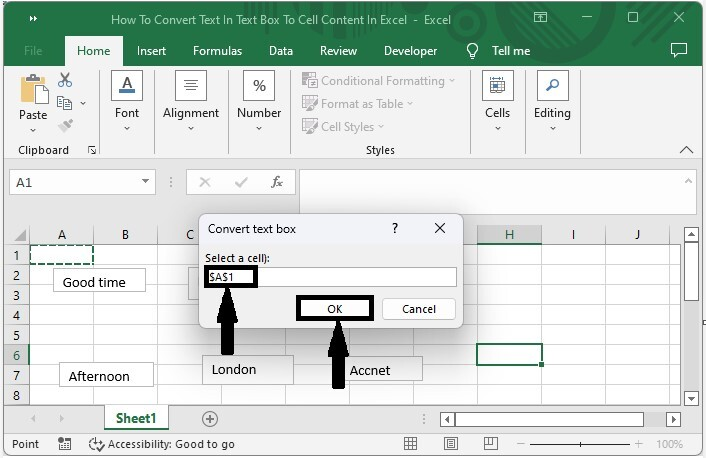# How to Convert Text in Text Box to Cell Content in Excel?

When we have data in text boxes in Excel and need to manually extract the data from the box, it can be a time-consuming process. We can’t perform any operations on the dates if they are in a text box, as they are treated as comments.

Read this tutorial to learn how you can convert text in a textbox to cell content in Excel. We can complete the process using the VBA application, as it can't be completed directly in Excel.

## Converting Text in Text Box to Cell Content in Excel

Here we will first insert the VBA module then run it to complete the task. Let's go over a simple procedure for converting text in a text box to cell content in Excel.

### Step 1

Let us consider any Excel sheet where it contains text boxes as similar to the data shown in the below image.Now right-click on the sheet name and select view code to open the VBA application, then click on inset and select module.

Right click > View code > Insert > Module

### Step 2

Copy the following program code in the textbox as shown in the below image.

### Program

Sub TextboxesToCell()
'Update By Nirmal
Dim xRg As Range
Dim xRow As Long
Dim xCol As Long
Dim xTxtBox As TextBox
Set xRg = Application.InputBox("Select a cell):", "Convert text box", _ActiveWindow.RangeSelection.AddressLocal, , , , , 8)
xRow = xRg.Row
xCol = xRg.Column
For Each xTxtBox In ActiveSheet.TextBoxes
Cells(xRow, xCol).Value = xTxtBox.Text
xTxtBox.Delete
xRow = xRow + 1
Next
End Sub### Step 3

Save the sheet as a macro-enabled workbook, click F5 to run the code, select the cell from where you want to start the list, and click OK.

Save > F5 > Cell > OK## Conclusion

In this tutorial, we used a simple example to demonstrate how you can convert text in a textbox to cell content in Excel.